International
Tables for
Crystallography
Volume F
Crystallography of biological macromolecules
Edited by M. G. Rossmann and E. Arnold

International Tables for Crystallography (2006). Vol. F, ch. 11.2, pp. 213-214   | 1 | 2 |

## Section 11.2.5. Integration by simple summation

A. G. W. Lesliea*

aMRC Laboratory of Molecular Biology, Hills Road, Cambridge CB2 2QH, England
Correspondence e-mail: andrew@mrc-lmb.cam.ac.uk

### 11.2.5. Integration by simple summation

| top | pdf |

#### 11.2.5.1. Determination of the best background plane

| top | pdf |

The background plane constants a, b, c are determined by minimizing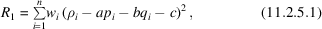where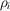is the total counts at the pixel with coordinates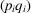with respect to the centre of the measurement box, and the summation is over the n background pixels.is a weight which should ideally be the inverse of the variance of. Assuming that the variance is determined by counting statistics, this gives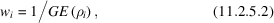where G is the gain of detector, which converts pixel counts to equivalent X-ray photons, and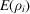is the expectation value of the background counts. In practice, the variation in background across the measurement box is usually sufficiently small that all weights can be considered to be equal.

This gives the following equations for a, b and c, as given in Rossmann (1979),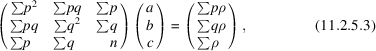where all summations are over the n background pixels.

#### 11.2.5.1.1. Outlier rejection

| top | pdf |

It is not unusual for the diffraction pattern to display features other than the Bragg diffraction spots from the crystal of interest. Possible causes are the presence of a satellite crystal or twin component, white-radiation streaks, cosmic rays or zingers. In order to minimize their effect on the determination of the background plane constants, the following outlier rejection algorithm is employed:

 (1) Determine the background plane constants using a fraction (say 80%) of the background pixels, selecting those with the lowest pixel values. (2) Evaluate the fit of all background pixels to this plane, rejecting those that deviate by more than three standard deviations. (3) Re-determine the background plane using all accepted pixels. (4) Re-evaluate the fit of all accepted pixels and reject outliers. If any new outliers are found, re-determine the plane constants.

The rationale for using a subset of the pixels with the lowest pixel values in step (1)is that the presence of zingers or cosmic rays, or a strongly diffracting satellite crystal, can distort the initial calculation of the background plane so much that it becomes difficult to identify the true outliers. Such features will normally only affect a small percentage of the background pixels and will invariably give higher than expected pixel counts. Selecting a subset with the lowest pixel values will facilitate identification of the true outliers. The initial bias in the resulting plane constant c due to this procedure will be corrected in step (3). Poisson statistics are used to evaluate the standard deviations used in outlier rejection, and the standard deviation used in step (2)is increased to allow for the choice of background pixels in step (1).

#### 11.2.5.2. Evaluating the integrated intensity and standard deviation

| top | pdf |

The summation integration intensity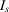is given by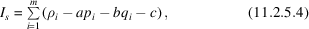where the summation is over the m pixels in the peak region of the measurement box. If the peak region has mm symmetry, this simplifies to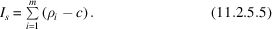To evaluate the standard deviation, this can be written as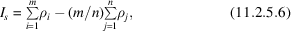where the second summation is over the n background pixels.

The variance inis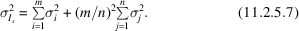From Poisson statistics this becomes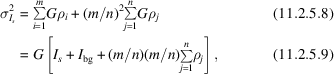where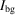is the background summed over all peak pixels. We can also write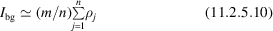(this is only strictly true if the background region has mm symmetry). Then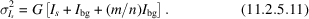This expression shows the importance of the background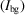in determining the standard deviation of the intensity. For weak reflections, the Bragg intensity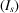is often much smaller than the background, and the error in the intensity is determined entirely by the background contribution.

#### 11.2.5.3. The effect of instrument or detector errors

| top | pdf |

Standard-deviation estimates calculated using (11.2.5.11) are generally in quite good agreement with observed differences between the intensities of symmetry-related reflections for weak or medium intensities. This is particularly true if other sources of systematic error are minimized by measuring the same reflections five or more times, by doing multiple exposures of the same small oscillation range and then processing the data in space group P1. However, even in this latter case, the agreement between strong intensities is significantly worse than that predicted using equation (11.2.5.11). This is consistent with the observation that it is very unusual to obtain merging R factors lower than 0.01, even for very strong reflections where Poisson statistics would suggest merging R factors should be in the range 0.002–0.003.

An experiment in which a diffraction spot recorded on photographic film was scanned many times on an optical microdensitometer showed that the r.m.s. variation in individual pixel values between the scans was greatest for those pixels immediately surrounding the centre of the spot, where the gradient of the optical density was greatest. One explanation for this observation is that these optical densities will be most sensitive to small errors in positioning the reading head, due to vibration or mechanical defects. A simple model for the instrumental contribution to the standard deviation of the spot intensity is obtained by introducing an additional term for each pixel in the spot peak: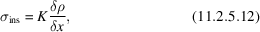where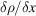is the average gradient and K is a proportionality constant. Taking a triangular reflection profile, the gradient and integrated intensity are related by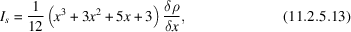where x is the half-width of the reflection (in pixels).

Writing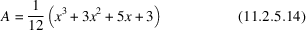gives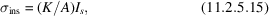where the factor A allows for differences in spot size and K is, ideally, a constant for a given instrument.

The total variance in the integrated intensity is then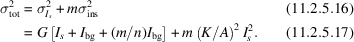A value for K can be determined by comparing the goodness-of-fit of the standard profiles to individual reflection profiles (of fully recorded reflections) with that calculated from combined Poisson statistics and the instrument error term. Standard deviations estimated using (11.2.5.17) give much more realistic estimates than those based on (11.2.5.11), even for data collected with charge-coupled-device (CCD) detectors where the physical model for the source of the error is clearly not appropriate.

### References

Rossmann, M. G. (1979). Processing oscillation diffraction data for very large unit cells with an automatic convolution technique and profile fitting. J. Appl. Cryst. 12, 225–238.Google Scholar